# Beggs and Robinson Oil Viscosity correlation

Jump to: navigation, search

## Beggs and Robinson Oil Viscosity correlation

Beggs and Robinson is an empirical correlation for the oil viscosity published in 1975 .

## Math & Physics

Dead oil viscosity: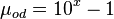$\mu_{od} = 10^x-1$

where: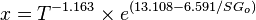$x = T^{-1.163} \times e^{(13.108-6.591/SG_{o})}$

Saturated oil viscosity (P < Pb):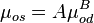$\mu_{os} = A \mu_{od}^B$

where: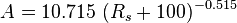$A = 10.715\ (R_s + 100)^{-0.515}$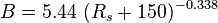$B = 5.44\ (R_s + 150)^{-0.338}$

Undersaturated oil viscosity (P > Pb):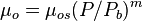$\mu_{o} = \mu_{os} (P/P_b)^m$

where: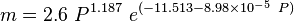$m = 2.6\ P^{1.187}\ e^{(-11.513-8.98 \times 10^{-5}\ P)}$

## Example. Calculation of the oil viscosity

Example source 

### Input data$T$ = 137 F°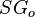$SG_o$ = 0.922 or 22 API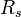$R_s$ = 90 scf/stb

Calculate the saturated oil viscosity?

### Solution

x = 1.2658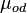$\mu_{od}$ = 17.44 cP

A = 0.719 B = 0.853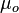$\mu_o$ = 8.24 cP

The solution is available in the online PVT calculator software model at www.pengtools.com

## Application range

Description of the Data Used: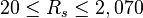$20 \le R_s \le 2,070$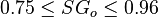$0.75 \le SG_o \le 0.96$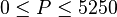$0 \le P \le 5250$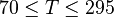$70 \le T \le 295$

Number of oil systems = 600
Number of dead oil observations = 460
Number of live oil observations = 2,073

## Nomenclature$A$ = coefficient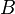$B$ = coefficient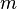$m$ = coefficient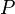$P$ = pressure, psia$R_s$ = solution gas-oil ratio, scf/stb$SG_o$ = oil specific gravity, dimensionless$T$ = temperature, °F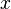$x$ = coefficient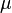$\mu$ = viscosity, cP

#### Subscripts

b - bubble point
od - dead oil
os - saturated oil
o - undersaturated oil